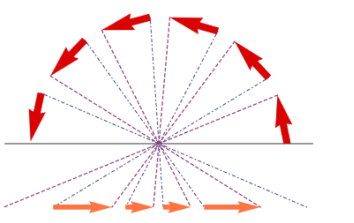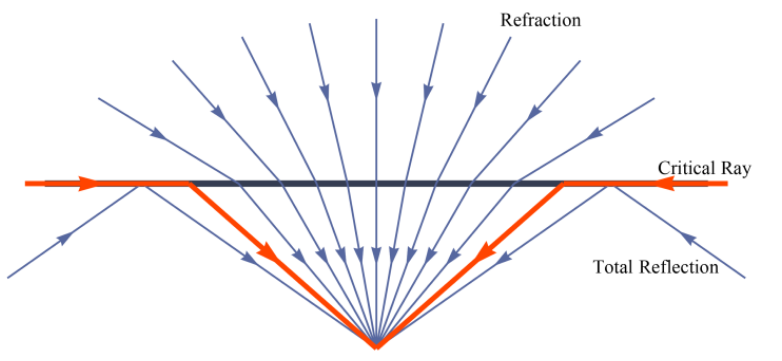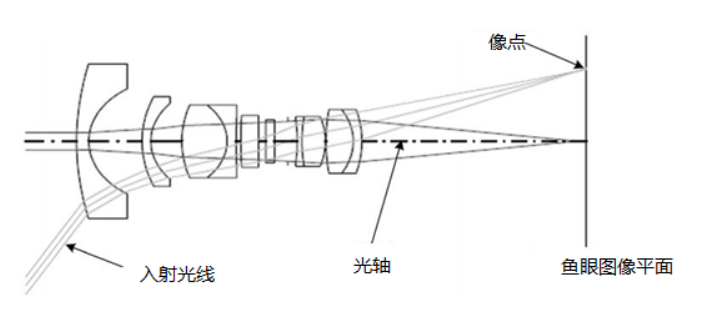# 1. 鱼眼镜头原理# 2. 鱼眼镜头模型1. 三维空间点线性地投影到一个球面上，它是一个虚拟的单位球面，它的球心与相机坐标系的原点重合
2. 单位球面上的点投影到图像平面上，这个过程是非线性的

• 等距投影 $r_d = f \theta$
• 等立体角投影 $r_d = 2fsin(\frac{\theta}{2})$
• 正交投影 $r_d = fsin( \theta)$
• 体视投影 $r_d = 2ftan(\frac{\theta}{2})$